# More plotting tools from nilearn#

In this example, we show how to use some plotting options available with plotting functions of nilearn. These techniques are essential for visualizing brain image analysis results.

Plotting functions of Nilearn, such as `plot_stat_map`, have a few useful parameters which control what type of display object will be returned, as well as how many cuts will be shown for example.

As we will see in the first part of this example, depending on the values of the parameters `display_mode` and `cut_coords`, plotting functions return different display objects, all subclasses of `nilearn.plotting.displays.OrthoSlicer`.

These objects implement various methods to interact with the figures. In the second part of this example, we show how to use these methods to further customize the figures obtained with plotting functions. More precisely, we will show how to use `add_edges`, `add_contours`, and `add_markers`, all essential in visualizing regions of interest images, or mask images overlaying on subject specific anatomical / EPI image.

The parameter `display_mode` is used to draw brain slices along given specific directions, where directions can be one of ‘ortho’, ‘tiled’, ‘mosaic’, ‘x’, ‘y’, ‘z’, ‘yx’, ‘xz’, ‘yz’. Whereas parameter `cut_coords` is used to specify a limited number of slices to visualize along given specific slice direction.

The parameter `cut_coords` can also be used to draw the specific cuts in the slices by giving its particular coordinates in MNI space accordingly with particular slice direction. This helps us point to the activation specific location of the brain slices.

See Plotting brain images for more details.

## First, we retrieve data from nilearn provided (general-purpose) datasets#

```from nilearn import datasets

# haxby dataset to have anatomical image, EPI images and masks
haxby_dataset = datasets.fetch_haxby()
haxby_anat_filename = haxby_dataset.anat
haxby_func_filename = haxby_dataset.func

# localizer dataset to have contrast maps
stat_img = motor_images.images
```

Now, we show from here how to visualize the retrieved datasets using plotting tools from nilearn.

```from nilearn import plotting
```

## Visualizing in - ‘sagittal’, ‘coronal’ and ‘axial’ with given coordinates#

The first argument `stat_img` is a path to the filename of a contrast map. The optional argument `display_mode` is given as a string ‘ortho’ to visualize the map in three specific directions xyz. Because of this, the plotting function returns a `OrthoSlicer` object. The optional `cut_coords` argument is specified here as a list of integers representing coordinates of each slice in the order [x, y, z]. By default the `colorbar` argument is set to `True` in `plot_stat_map`.

```plotting.plot_stat_map(stat_img, display_mode='ortho',
cut_coords=[36, -27, 60],
title="display_mode='ortho', cut_coords=[36, -27, 60]")
``````<nilearn.plotting.displays._slicers.OrthoSlicer object at 0x7f12396018d0>
```

## Visualizing in - single view ‘axial’ with number of cuts=5#

For axial visualization, we set `display_mode='z'`. As a consequence `plot_stat_map` returns a `ZSlicer` object. The parameter `cut_coords` is provided here as an integer (5) rather than a list, which implies that the number of cuts in the slices should be 5 maximum. Note that the coordinates used to cut the slices are selected automatically.

```plotting.plot_stat_map(stat_img, display_mode='z', cut_coords=5,
title="display_mode='z', cut_coords=5")
```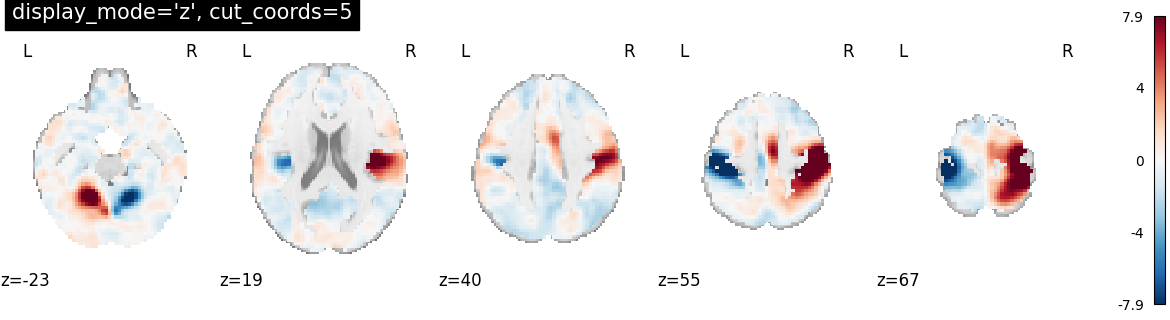```<nilearn.plotting.displays._slicers.ZSlicer object at 0x7f1240f83880>
```

## Visualizing in - single view ‘sagittal’ with only two slices#

For sagittal visualization, we set `display_mode='x'` which returns a `XSlicer` object. Additionally, we provide the coordinates of the slices as a list of integers.

```plotting.plot_stat_map(stat_img, display_mode='x',
cut_coords=[-36, 36],
title="display_mode='x', cut_coords=[-36, 36]")
```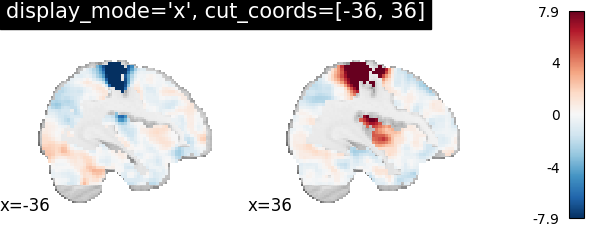```<nilearn.plotting.displays._slicers.XSlicer object at 0x7f123a81a7a0>
```

## Visualizing in - ‘coronal’ view with single cut#

For coronal view, we set `display_mode='y'` which returns a `YSlicer` object. `cut_coords` is provided as an integer (1), and the coordinates are, again, selected automatically.

```plotting.plot_stat_map(stat_img, display_mode='y', cut_coords=1,
title="display_mode='y', cut_coords=1")
```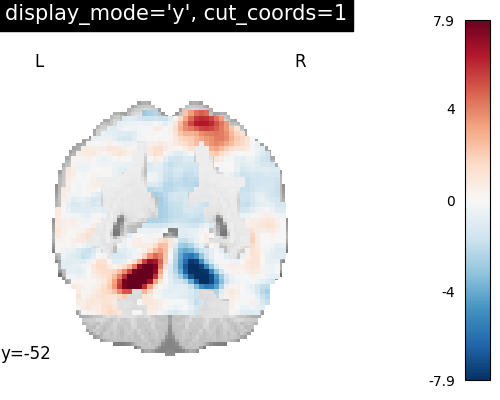```<nilearn.plotting.displays._slicers.YSlicer object at 0x7f124a13d210>
```

## Visualizing without a colorbar on the right side#

The argument `colorbar` should be set to `False` to show plots without a colorbar on the right side.

```plotting.plot_stat_map(stat_img, display_mode='z',
cut_coords=1, colorbar=False,
title="display_mode='z', cut_coords=1, colorbar=False")
```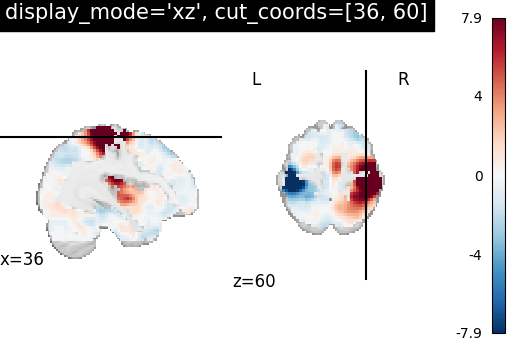```<nilearn.plotting.displays._slicers.ZSlicer object at 0x7f123a818dc0>
```

## Visualize in - two views ‘sagittal’ and ‘axial’ with given coordinates#

In order to visualize both sagittal and axial views, we set `display_mode='xz'`, where ‘x’ stands for sagittal and ‘z’ for axial view. Function `plot_stat_map` thus returns a `XZSlicer` object. Finally, the argument `cut_coords` should match with the input number of views (two here). It is provided as a list of integers here to select the slices to be displayed.

```plotting.plot_stat_map(stat_img, display_mode='xz',
cut_coords=[36, 60],
title="display_mode='xz', cut_coords=[36, 60]")
```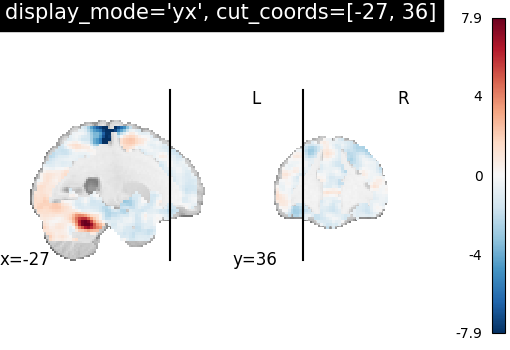```<nilearn.plotting.displays._slicers.XZSlicer object at 0x7f124185ceb0>
```

## Changing the views to ‘coronal’, ‘sagittal’ views with coordinates#

Similarly, we can set `display_mode='yx'` for combining a coronal with a sagittal view, which will return a `YXSlicer` object. The coordinates will be assigned in the order of direction as [x, y, z].

```plotting.plot_stat_map(stat_img, display_mode='yx',
cut_coords=[-27, 36],
title="display_mode='yx', cut_coords=[-27, 36]")
``````<nilearn.plotting.displays._slicers.YXSlicer object at 0x7f123a8196c0>
```

## Now, views are changed to ‘coronal’ and ‘axial’ views with coordinates#

We can set `display_mode='yz'` to combine a coronal with an axial view, which will return a `YZSlicer` object.

```plotting.plot_stat_map(stat_img, display_mode='yz',
cut_coords=[-27, 60],
title="display_mode='yz', cut_coords=[-27, 60]")
```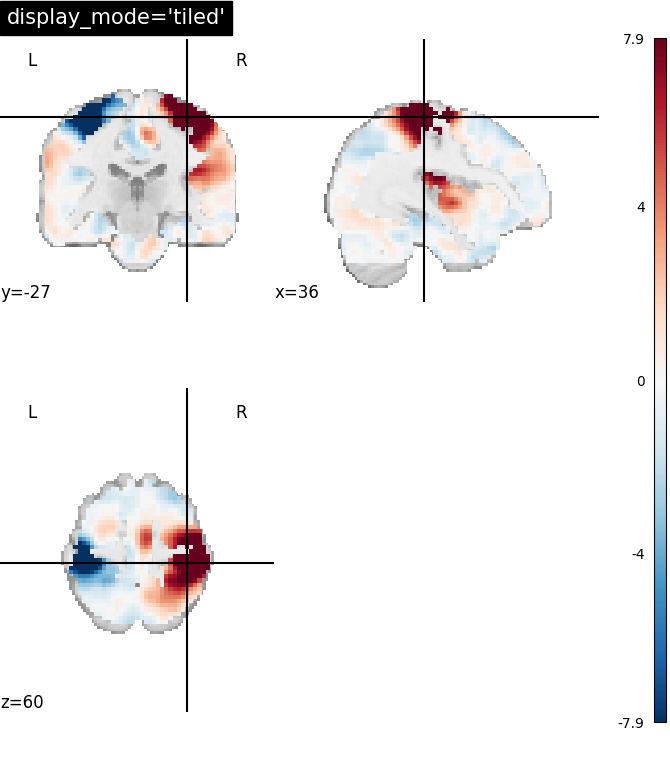```<nilearn.plotting.displays._slicers.YZSlicer object at 0x7f122cb2c0a0>
```

## Visualizing three views in 2x2 fashion#

If we want to combine three views in a 2x2 way, we can set `display_mode='tiled'`, which will combine sagittal, coronal, and axial views. In this case, `plot_stat_map` will return a `TiledSlicer` object.

```plotting.plot_stat_map(stat_img, display_mode='tiled',
cut_coords=[36, -27, 60],
title="display_mode='tiled'")
```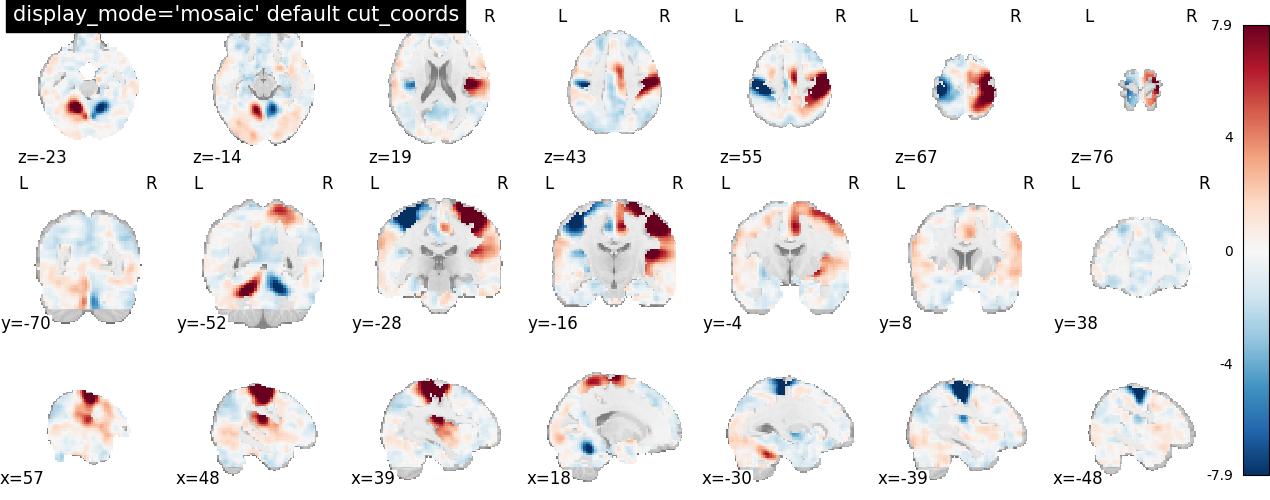```<nilearn.plotting.displays._slicers.TiledSlicer object at 0x7f123ced58a0>
```

## Visualizing three views along multiple rows and columns#

If we set `display_mode='mosaic'`, we can easily combine sagittal, coronal, and axial views with different rows and columns. In this situation, `plot_stat_map` returns a `MosaicSlicer` object. In addition, we show here the default option `cut_coords=None`.

```plotting.plot_stat_map(stat_img, display_mode='mosaic',
title="display_mode='mosaic' default cut_coords")
```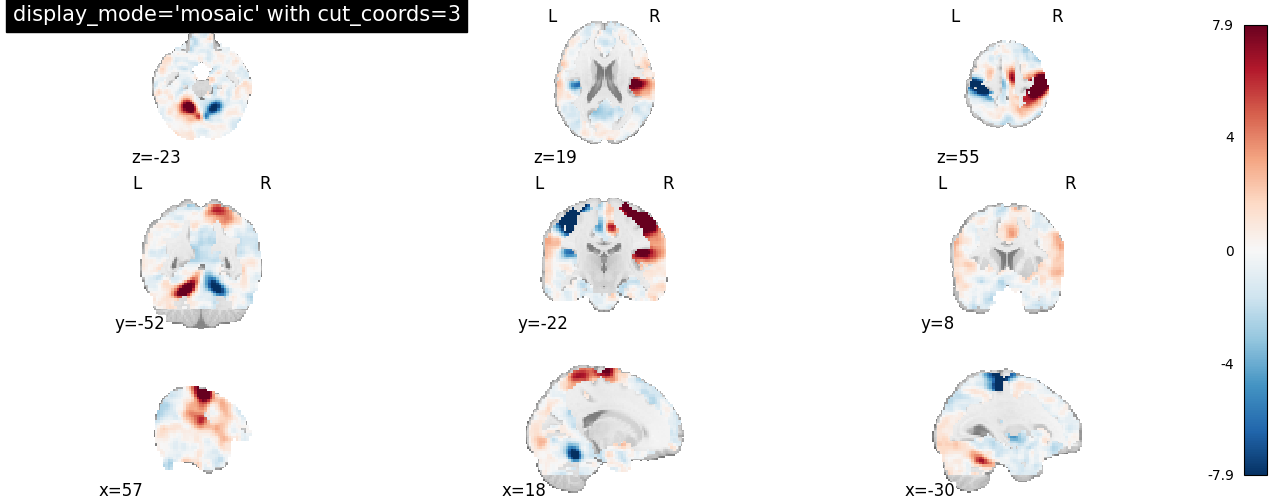```<nilearn.plotting.displays._slicers.MosaicSlicer object at 0x7f124185d870>
```

## Now, changing the number of slices along columns#

Here, we still set `display_mode='mosaic'`, but we set the number of slices to be the same across views. In this case, we can specify it as an integer, i.e. `cut_coords=3`.

```plotting.plot_stat_map(stat_img, display_mode='mosaic',
cut_coords=3,
title="display_mode='mosaic' with cut_coords=3")
```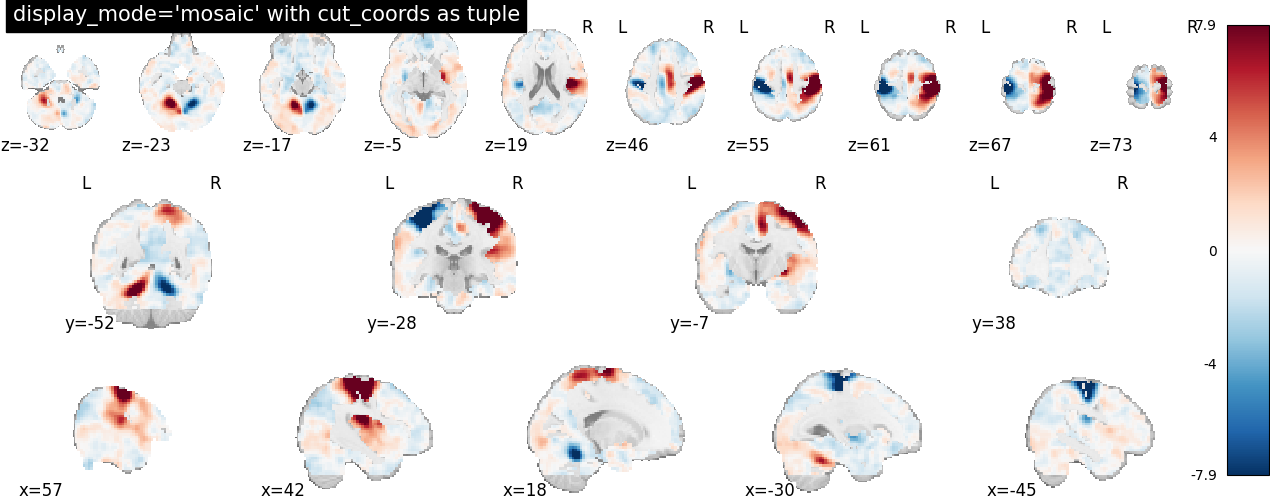```<nilearn.plotting.displays._slicers.MosaicSlicer object at 0x7f122cb36c20>
```

## Now, another way of limiting the number of slices along rows and columns#

It can be the case that we want to display a different number of cuts in each view. In this situation, we still set `display_mode='mosaic'`, but we specify the number of slices as a tuple of length 3.

```plotting.plot_stat_map(stat_img, display_mode='mosaic',
cut_coords=(5, 4, 10),
title="display_mode='mosaic' with cut_coords as tuple")
```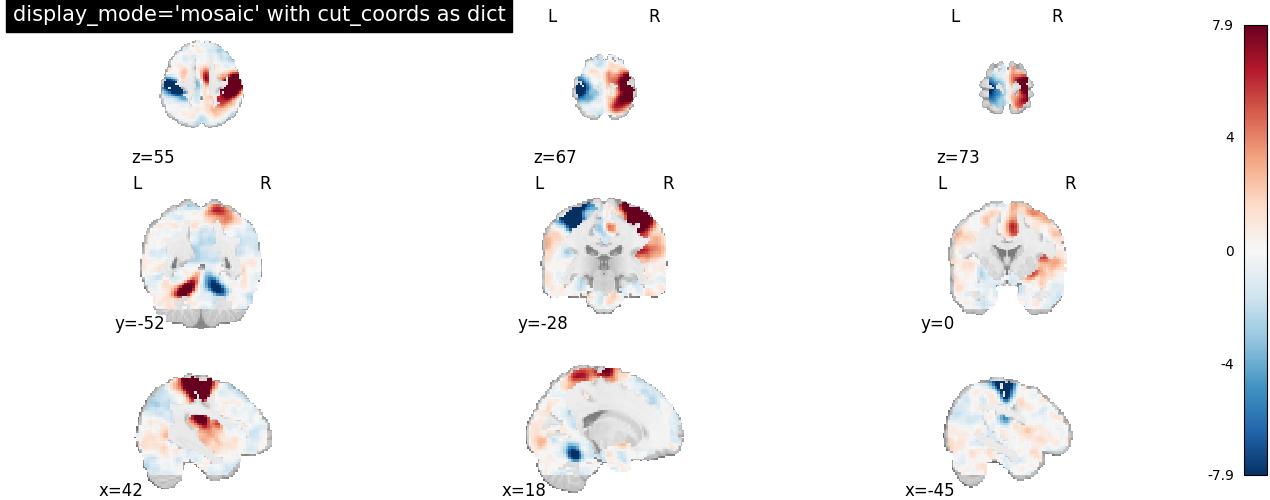```<nilearn.plotting.displays._slicers.MosaicSlicer object at 0x7f124156b160>
```

## Demonstrating various display features#

In this second part, we demonstrate how to interact with the obtained figures. More precisely, we will show how to use specific methods of the display objects which can be helpful in projecting brain imaging results for further interpretation.

```# Import image processing tool for basic processing of functional brain image
from nilearn import image

# Compute voxel-wise mean functional image across time dimension. Now we have
# functional image in 3D assigned in mean_haxby_img
mean_haxby_img = image.mean_img(haxby_func_filename)
```

## Showing how to use add_edges#

Now let us see how to use the method `add_edges` for checking coregistration by overlaying anatomical image as edges (red) on top of mean functional image (background), both being of same subject.

First, we call the `nilearn.plotting.plot_anat` plotting function, with a background image as first argument, in this case the mean fMRI image.

We then use the `add_edges` method. The first argument is the anatomical image and, by default, edges will be displayed in red (‘r’). To choose a different color, use the `color` argument.

```display = plotting.plot_anat(mean_haxby_img, title="add_edges")
```## How to use add_contours#

Here, we show how to plot the outline of a mask (in red) on top of the mean EPI image with the method `add_contours`. This method is useful for region specific interpretation of brain images

As before, we call the `plot_anat` function with a background image as first argument, in this case the mean fMRI image, and argument `cut_coords` as a list for manual cuts with coordinates pointing at masked brain regions.

We then use the `add_contours` method of the display object returned by `plot_anat`. We provide the path to a mask image from the Haxby dataset as the first argument, and we provide `levels` as a list of values to select particular levels in the contour to display. We also specify `colors='r'` to display edges in red (See function `contour` to use more options).

```display = plotting.plot_anat(mean_haxby_img, title="add_contours",
cut_coords=[-34, -39, -9])

```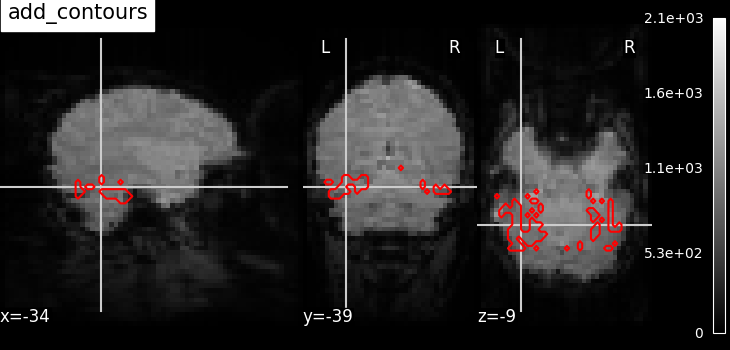Here, we plot the outline of the mask (in blue) with color fillings using the same method `add_contours`.

By default, no color fillings will be shown using `add_contours`. To see contours with color fillings, use argument `filled=True`. Here, contour colors are changed to blue ‘b’, and we specify `alpha=0.7` to set the transparency of the fillings. See function `contourf` to use more options (given that `filled` should be `True`).

```display = plotting.plot_anat(mean_haxby_img,
cut_coords=[-34, -39, -9])

levels=[0.5], colors='b')
```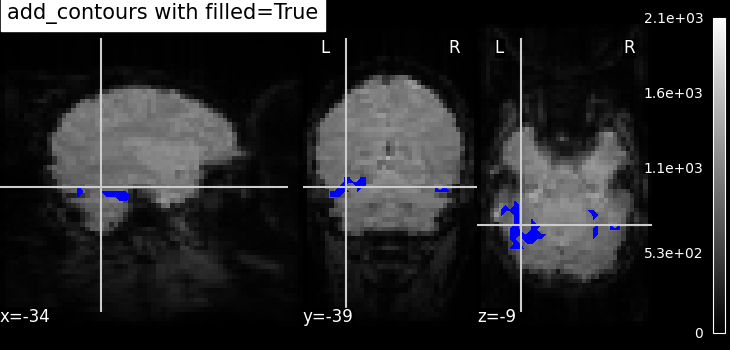```/home/yasmin/nilearn/nilearn/nilearn/plotting/displays/_axes.py:71: UserWarning:

No contour levels were found within the data range.
```

## Plotting seeds using add_markers#

Plotting seed regions of interest as spheres using method `add_markers` with MNI coordinates of interest.

The coordinates of the seed regions should be specified as the first argument, and second argument `marker_color` is used to denote the color of the sphere (in this case yellow ‘y’). The third argument `marker_size` is used to control the size of the sphere.

```display = plotting.plot_anat(mean_haxby_img, title="add_markers",
cut_coords=[-34, -39, -9])
coords = [(-34, -39, -9)]
```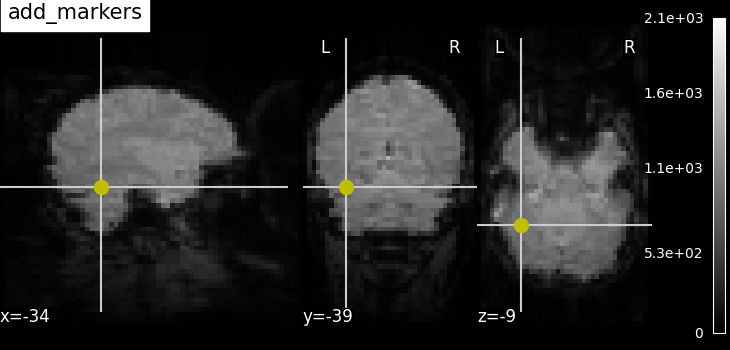## Annotating plots#

It is possible to alter the default annotations of plots, using the method `annotate` of the display objects. For example, we can add a scale bar at the bottom right of each view:

```display = plotting.plot_anat(mean_haxby_img,
title="adding a scale bar",
cut_coords=[-34, -39, -9])
display.annotate(scalebar=True)
```Further configuration can be achieved by setting `scale_*` keyword args. For instance, we can change the `units` to `mm`, or use a different scale bar size.

```display = plotting.plot_anat(mean_haxby_img,
title="adding a scale bar",
cut_coords=[-34, -39, -9])
display.annotate(scalebar=True, scale_size=25, scale_units='mm')
```## Saving plots to file#

Finally, we can save a plot to file in two different ways:

First, we can save the contrast maps plotted with the function `nilearn.plotting.plot_stat_map` using the built-in parameter `output_file`. We provide the filename and the file extension as a string (supported extensions are .png, .pdf, .svg).

```plotting.plot_stat_map(stat_img,
title='Using plot_stat_map output_file',
output_file='plot_stat_map.png')
```

A second way to save plots is by using the method `savefig` of the display object returned.

```display = plotting.plot_stat_map(stat_img,
title='Using display savefig')

display.savefig('plot_stat_map_from_display.png')

# In non-interactive settings make sure you close your displays
display.close()

plotting.show()
```

Total running time of the script: ( 0 minutes 51.463 seconds)

Estimated memory usage: 913 MB

Gallery generated by Sphinx-Gallery